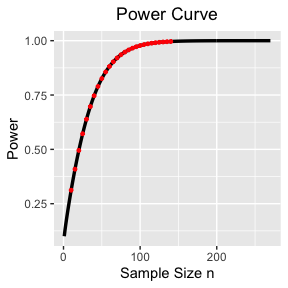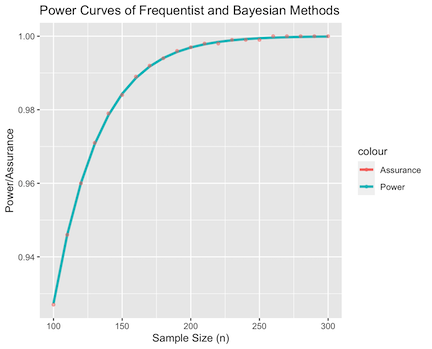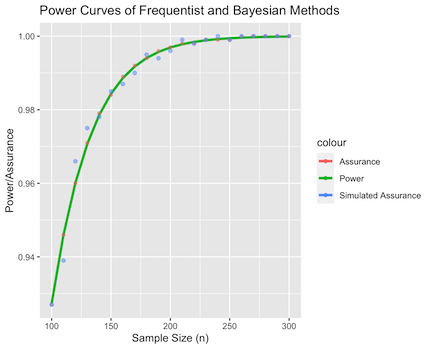# bayesassurance: Functions Based on Closed-Form Solutions

This vignette is intended to demonstrate non-simulation based features of the bayesassurance package. The primary focus of this tutorial involves discussing the underlying closed-form solutions embedded in the pwr_freq() and assurance_nd_na() functions followed by examples on how these functions are implemented in R. This document will also cover add-on tools and features that many of the simulation-based functions are contingent upon. In particular, the matrix generating functions, gen_Xn() and gen_Xn_longitudinal(), will be relevant in the next set of vignettes.

# 1 The pwr_freq() Function

The pwr_freq() function takes in a set of fixed inputs pertaining to a one-sample hypothesis test and returns the corresponding statistical power of the test.

To elaborate, consider the following one-sided hypothesis test for population mean $$\theta$$: $H_0: \theta = \theta_0 \\ H_a: \theta = \theta_1 > \theta_0,$ where $$\theta_0$$ is the reference value that we will test against. Assuming the known population variance is denoted as $$\sigma^2$$ and the sample mean’s distribution is approximately Gaussian, $$H_0$$ is rejected if $$\bar{y} > \theta_0 + \frac{\sigma}{\sqrt{n}}Z_{1-\alpha}$$, where $$\bar{y}$$ is the sample mean, $$\alpha$$ is the specified Type I error, $$Z_{1-\alpha}$$ is the corresponding quantile of the Gaussian distribution, and $$n$$ is the sample size. We can use this rejection criteria to derive the statistical power of the test, defined by $$1-\beta = P(\text{reject } H_0 | H_a \text{ is true})$$. Straightforward standardization procedure leads to $\begin{equation} \label{eq:power_func} 1-\beta = P\left(\bar{y} > \theta_0 + \frac{\sigma}{\sqrt{n}}Z_{1-\alpha} \Bigg| \theta = \theta_1 \right) = \Phi\left(\sqrt{n}\frac{\Delta}{\sigma} - Z_{1-\alpha}\right), \end{equation}$ where $$\Delta = \theta_1 - \theta_0$$ denotes the critical difference and $$\Phi$$ denotes the cumulative distribution function of the standard normal. Similar steps can be taken to determine the power expressions for alternative comparisons of $$H_a$$. When $$H_a: \theta_1 < \theta_0$$, the power is determined as $1-\beta = P\left(\bar{y} < \theta_0 - \frac{\sigma}{\sqrt{n}}Z_{1-\alpha} \Bigg| \theta = \theta_1 \right) = 1 - \Phi\Big(\sqrt{n}\frac{\Delta}{\sigma} + Z_{1-\alpha}\Big).$ Finally in the two-sided case where $$H_a: \theta_1 \neq \theta_0$$, the power is determined as \begin{align*} 1-\beta &= P\left(\bar{y} < \theta_0 - \frac{\sigma}{\sqrt{n}}Z_{1-\alpha/2} \Bigg | \theta = \theta_1 \right) + P\left(\bar{y} > \theta_0 + \frac{\sigma}{\sqrt{n}}Z_{1-\alpha/2} \Bigg | \theta = \theta_1 \right)\\ &= 1 + \Phi\left(\sqrt{n}\frac{\Delta}{\sigma} - Z_{1-\alpha/2}\right) - \Phi\left(\sqrt{n}\frac{\Delta}{\sigma} + Z_{1-\alpha/2}\right). \end{align*}

## 1.1 Example

Load the bayesassurance package.

library(bayesassurance)

Specify the following inputs:

1. n: Sample size (either vector or scalar)
2. theta_0: Initial value the parameter is set equal to in the null hypothesis
3. theta_1: Alternative value to be compared to theta_0.
4. sigsq: Known variance $$\sigma^2$$
5. alt: Specifies comparison between theta_1 and theta_0 in the alternative hypothesis, where alt = "greater" tests if $$\theta_1 > \theta_0$$, alt = "less" tests if $$\theta_1 < \theta_0$$, and alt = "two.sided" performs a two-sided test for $$\theta_1 \neq \theta_0$$. alt is set to "greater" by default.
6. alpha: Significance level

As an example, we assign the following set of arbitrary inputs to pass into pwr_freq() and save the output as pwr_vals.

n <- seq(10, 140, 5)
pwr_vals <- bayesassurance::pwr_freq(n = n, theta_0 = 0.15, theta_1 = 0.35, sigsq = 0.3,
alt = "greater", alpha = 0.05)

The saved output pwr_vals contains two objects:

1. pwr_table: table of sample sizes and corresponding power values.
2. pwr_plot: power curve that is only returned if n is a vector.

To view the power curve, simply type pwr_vals$pwr_plot in the R console. To ensure a smooth continuous curve, the function determines additional power values for a wider range of sample sizes that surround the inputted values specified for n. The resulting power curve thus includes values above, below, and between the inputted values specified for n, with specific values of interest marked in red. The first six entries of the power table can be shown by calling pwr_vals$pwr_table.

head(pwr_vals$pwr_table) n Power 10 0.3120128 15 0.4087972 20 0.4952685 25 0.5717723 30 0.6387600 35 0.6968609 The power plot is produced using ggplot2, displaying the inputted sample sizes on the x-axis and the resulting power values on the y-axis. The points highlighted in red denote values specified by the user. In this example, we see red points marked along the values of n=10 through n=140 in increments of 5. pwr_vals$pwr_plotIf a scalar value is inputted into n, a single power value is returned.

n <- 20
pwr_freq(n = n, theta_0 = 0.15, theta_1 = 0.35, sigsq = 0.3,
alt = "greater", alpha = 0.05)
#>  "Power: 0.495"

# 2 The assurance_nd_na() Function

The assurance_nd_na() function determines the assurance of a specified outcome based on a closed-form solution that is derived under the Bayesian setting.

Suppose we seek to evaluate the tenability of $$\theta > \theta_0$$ given data from a Gaussian population with mean $$\theta$$ and known variance $$\sigma^2$$. We assign two sets of priors for $$\theta$$, one at the $$\textit{design stage}$$ and the other at the $$\textit{analysis stage}$$. The analysis objective specifies the condition that needs to be satisfied, which in this case, involves observing that $$P(\theta > \theta_0| \bar{y}) > 1-\alpha$$. The design objective seeks a sample size that is needed to ensure that the analysis objective is met $$100\delta\%$$ of the time, where $$\delta$$ denotes the assurance.

Let $$\theta \sim N\big(\theta_1, \frac{\sigma^2}{n_a}\big)$$ be our analysis stage prior and $$\theta \sim N\big(\theta_1, \frac{\sigma^2}{n_d}\big)$$ be our design stage prior, where $$n_a$$ and $$n_d$$ are precision parameters that respectively quantify the degree of belief carried towards parameter $$\theta$$ and the degree of belief carried towards the population from which we are drawing samples from to evaluate $$\theta$$. Then, given the likelihood $$\bar{y} \sim N\big(\theta, \frac{\sigma^2}{n}\big)$$, we can obtain the posterior distribution of $$\theta$$ by multiplying the analysis prior and likelihood: $\begin{equation}\label{eq: simple_posterior} N\left(\theta {\left | \theta_1, \frac{\sigma^2}{n_a} \right.}\right) \times N\left(\bar{y} {\left | \theta, \frac{\sigma^2}{n} \right.}\right) \propto N\left(\theta {\left | \frac{n_a}{n + n_a}\theta_1 + \frac{n}{n + n_a}\bar{y}, \frac{\sigma^2}{n + n_a}\right.}\right)\;. \end{equation}$

This posterior distribution gives us $$P(\theta > \theta_0 | \bar{y})$$ and the assurance is then defined as $\begin{equation}\label{eq:assurance} \delta = P_{\bar{y}}\left\{\bar{y}: P(\theta > \theta_0 | \bar{y}) > 1 - \alpha\right\}. \end{equation}$ The assurance expression can be expanded out further by using the marginal distribution of $$\bar{y}$$, which is obtained by $\int{N\left(\theta {\left|\theta_1, \frac{\sigma^2}{n_d}\right.}\right) \times N\left(\bar{y} {\left | \theta, \frac{\sigma^2}{n} \right.}\right) d\theta} = N\left(\bar{y} {\left |\theta_1, \Big(\frac{1}{n} + \frac{1}{n_d}\Big) \sigma^2 \right.}\right) .$ Since the assurance definition is conditioned on $$\bar{y}$$, we use this to standardize the assurance expression to obtain the following closed-form solution: $\delta(\Delta, n) = \Phi\Bigg(\sqrt{\frac{nn_d}{n+n_d}} \Bigg[\frac{n+n_a}{n}\frac{\Delta}{\sigma} + Z_{\alpha}\frac{\sqrt{n+n_a}}{n}\Bigg]\Bigg),$ where $$\Delta = \theta_1 - \theta_0$$. Similar steps can be taken to derive the closed-form expressions of assurance for $$\theta < \theta_0$$ and $$\theta \neq \theta_0$$. If we wish to seek the tenability of $$\theta < \theta_0$$, the assurance is given by $\delta(\Delta, n) = 1-\Phi\Bigg(\sqrt{\frac{nn_d}{n+n_d}} \Bigg[\frac{n+n_a}{n}\frac{\Delta}{\sigma} + Z_{1-\alpha}\frac{\sqrt{n+n_a}}{n}\Bigg]\Bigg).$ Finally, for evaluating the tenability of $$\theta \neq \theta_0$$, the assurance is given by $\delta(\Delta, n) = 1-\Phi\Bigg(\sqrt{\frac{nn_d}{n+n_d}} \Bigg[\frac{n+n_a}{n}\frac{\Delta}{\sigma} + Z_{1-\alpha/2}\frac{\sqrt{n+n_a}}{n}\Bigg]\Bigg) + \Bigg[\frac{n+n_a}{n}\frac{\Delta}{\sigma} + Z_{\alpha/2}\frac{\sqrt{n+n_a}}{n}\Bigg]\Bigg).$

## 2.1 Example

After loading in the bayesassurance package, specify the following inputs:

1. n: sample size (scalar or vector)
2. n_a: sample size at analysis stage that quantifies the amount of prior information we have for parameter $$\theta$$
3. n_d: sample size at design stage that quantifies the amount of prior information we have for where the data is being generated from.
4. theta_0: parameter value that is known a priori (typically provided by the client)
5. theta_1: alternative parameter value that will be tested in comparison to theta_0. See alt for specification options.
6. alt: specifies alternative test case, where alt = "greater" evaluates the tenability of $$\theta > \theta_0$$,alt = "less" evaluates the tenability of $$\theta < \theta_0$$, and alt = "two.sided" evaluates the tenability of $$\theta \neq \theta_0$$. alt is set to "greater" by default.
7. sigsq: known variance $$\sigma^2$$
8. alpha: significance level

Suppose we assign the following fixed parameters to determine the Bayesian assurance for the given vector of sample sizes.

n <- seq(10, 500, 5)
n_a <- 1e-8
n_d <- 1e+8
theta_0 <- 0.15
theta_1 <- 0.25
sigsq <- 0.104
assur_vals <- assurance_nd_na(n = n, n_a = n_a, n_d = n_d, theta_0 = theta_0,
theta_1 = theta_1, sigsq = sigsq, alt = "greater",
alpha = 0.05)

Just as with the pwr_freq() function, the assurance_nd_na() function returns a list of two outputs provided that the inputted sample size $$n$$ is a vector of values.

1. assurance_table: table of sample sizes and corresponding power values.
2. assurance_plot: assurance curve that is only returned if n is a vector.

The first six entries of the resulting assurance table is shown by calling assur_vals$assurance_table. head(assur_vals$assurance_table)
n Assurance
10 0.2532578
15 0.3285602
20 0.3981637
25 0.4623880
30 0.5213579
35 0.5752063

The assurance plot is produced using the ggplot2 package. It displays the inputted sample sizes on the x-axis and the resulting assurance values on the y-axis. Similar to pwr_freq(), the assurance_nd_na() function determines additional assurance values for a wider range of sample sizes that surround the inputted values specified for n. The resulting assurance curve thus includes values above, below, and between the inputted values specified for n, with specific values of interest marked in red.

assur_vals$assur_plot # 3 The pwr_curves() Function The pwr_curves() function constructs a single plot based on the combined set of inputs used to compute the frequentist power and Bayesian assurance. The plot includes three curves that include the power curve, the assurance curve obtained under a closed-form solution, and optionally, the assurance curve obtained under a simulation setting. The optional simulated assurance points are obtained using bayes_sim, which is discussed in a later vignette. Load in the bayesassurance package and specify the following parameters: 1. n: sample size (either scalar or vector) 2. n_a: Precision parameter that quantifies the degree of belief carried towards parameter $$\theta$$ 3. n_d: Precision parameter that quantifies the degree of belief carried towards the population from which we are drawing samples from to evaluate $$\theta$$. 4. theta_0: parameter value that is known a priori (typically provided by the client) 5. theta_1: alternative parameter value that will be tested in comparison to #’ theta_0. See alt for specification options. 6. sigsq: known variance $$\sigma^2$$ 7. alt: specifies alternative test case, where alt = "greater" tests if $$\theta > \theta_0$$, alt = "less" tests if $$\theta < \theta_0$$, and alt = "two.sided" performs a two-sided test. By default, alt = "greater". 8. alpha: significance level 9. bayes_sim: when set to TRUE, this indicates that the user wants to include simulated assurance results in the outputted plot. Default setting is FALSE. 10. mc_iter: If bayes_sim = TRUE, specifies the number of MC samples to evaluate. Default set to 5000 when not specified. ## 3.1 Examples Example 1 The following code chunk has bayes_sim set to FALSE, outputting just the power and assurance curves obtained from pwr_freq() and assurance_nd_na(). n <- seq(100, 300, 10) n_a <- 1e-8 n_d <- 1e+8 theta_0 <- 0.15 theta_1 <- 0.25 sigsq <- 0.104 alpha <- 0.05 out1 <- bayesassurance::pwr_curve(n, n_a, n_d, theta_0, theta_1, sigsq, alt = "greater", alpha, bayes_sim = FALSE) Three objects are returned as a list in out1. They are 1. power_table: table of sample sizes and corresponding power values. 2. assurance_table: table of sample sizes and corresponding assurance values 3. plot: figure displaying power curve and assurance values Similar to previous examples, the first six entries of the resulting power table is shown by calling out1$power_table.

head(out1$power_table) n Power 100 0.9273057 110 0.9460128 120 0.9601112 130 0.9706665 140 0.9785223 150 0.9843375 Likewise, the first six entries of the resulting assurance table is shown by calling out1$assurance_table, whose results are equal to those of the power table.

head(out1$assurance_table) n Assurance 100 0.9273056 110 0.9460127 120 0.9601111 130 0.9706664 140 0.9785222 150 0.9843374 The resulting plot is displayed by calling out1$plot.Example 2

The next code chunk has bayes_sim set to TRUE, outputting the assurance values obtained by sampling from the posterior distribution using bayes_sim() in addition to the power and assurance curves obtained from pwr_freq() and assurance_nd_na()

n <- seq(100, 300, 10)
n_a <- 1e-8
n_d <- 1e+8
theta_0 <- 0.15
theta_1 <- 0.25
sigsq <- 0.104
alpha <- 0.05

set.seed(10)
out2 <- bayesassurance::pwr_curve(n, n_a, n_d, theta_0, theta_1, sigsq, alt = "greater",
alpha, bayes_sim = TRUE)

Just as we have done in the previous example, the first six entries of the resulting assurance table from the bayes_sim() function is displayed by calling out2$bayes_sim_table. head(out2$bayes_sim_table)
n Assurance
100 0.9292
110 0.9492
120 0.9562
130 0.9724
140 0.9782
150 0.9846

The resulting plot is displayed by calling out2$plot. out2$plot